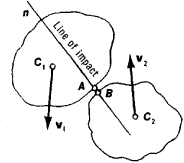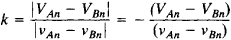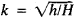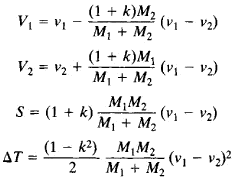# Impact

(redirected from impacts)
Also found in: Dictionary, Thesaurus, Medical, Legal, Acronyms, Idioms.
Related to impacts: Environmental impacts

## Impact

A force, also known as impulsive force, which acts only during a short time interval but which is sufficiently large to cause an appreciable change in the momentum of the system on which it acts. The momentum change produced by the impulsive force is described by the momentum-impulse relation. See Collision (physics), Impulse (mechanics)

## Impact

a forceful collision between two bodies. In English the term “impact” is also sometimes applied to the impulsive forces acting in collisions. The concept of impact need not be restricted to the collision of solid bodies. For example, we may speak of the impact of a jet of fluid on a solid body and the impact of a solid body on the surface of a liquid. The Russian term for impact—udar—also has the meaning of shock and consequently is applied to a number of additional phenomena, such as a water hammer and the action of an explosion or shock wave on a body.

The interval of time during which an impact occurs is small and usually ranges from several ten thousandths to a few millionths of a second. The impulsive forces acting at the areas of contact of colliding bodies, however, are very large. These forces vary within broad limits during the time of impact and reach values such that the average pressure (stresses) at the areas of contact is of the order of 104 or even 105 kilograms-force/cm2 (1 kilogram-force/cm2 = 105 newtons/m2).

During the time of contact, the action of the impulsive forces causes a substantial change in the velocities of the points of a body. Other possible consequences of impact include permanent deformation, the production of sound waves, the heating of the bodies, and the occurrence of changes in the mechanical properties of the materials composing the bodies. When the impact speed exceeds its critical value, a body will fracture at the place of impact. The critical speeds for metals range from about 15 m/sec, in the case of copper, to about 150 m/sec or more, in the case of high-grade steels.

The change in the velocities of points of a body during impact can be determined by methods of the general theory of impact. As a measure of the mechanical interaction of bodies during impact, these methods use not the impulsive force P but the impact impulse S, which is the impulse of the force during the time of impact T. Since T is small, the impulses of all external forces (such as gravity) and the displacements of the points of the body during the impact are disregarded. The basic equations of the general theory of impact follow from theorems on the change of the momentum and angular momentum of a system in a collision. The velocities after the impact can be found by means of these theorems when the applied impact impulse and the velocities before impact are known. If the body is not free, the impulsive constraints can also be found.

When two bodies collide, the collision can be divided into two periods. The first period begins at the instant that points A and B of the bodies (see Figure 1) come into contact. At this instant their rate of approach is vAnvBn, where vAn and vBn are theFigure 1. Impact between two bodies

components of the velocities vA and vB along n, the common normal to the surfaces of the bodies at A and B. This normal is known as the line of impact. By the end of the first period, the bodies have ceased moving toward each other, and part of their kinetic energy has been transformed into potential energy of deformation. In the second period, the reverse transformation occurs: the potential energy of elastic deformation is transformed into kinetic energy of the bodies, which are now separating. At the end of the second period, points A and B are moving apart at the rate VAnVBn. If the bodies are perfectly elastic, the mechanical energy is completely restored by the end of the second period, and ǀVAnVBn = ǀvAnvBnǀ. On the other hand, the impact of perfectly inelastic bodies ends with the first period; in this case, VAnVBn = 0.

When real bodies collide, the mechanical energy is only partially restored by the time the impact ends. The rest of the energy is lost as a result of the permanent deformation of the bodies, the heating of the bodies, and so on. Thus, ǀVAnVBnǀ < ǀvAnvBnǀ. The losses are calculated by introducing the coefficient of restitution k, which is assumed to depend only on the physical properties of the materials of the bodies:In the case of impact involving a stationary body, VBn = vBn = 0 and k = – VAn/vAn. The value of k is determined experimentally. One method is to measure the height h reached by a ball in its rebound after falling freely onto a horizontal plate from a height H; in this case,. Experimental data yield the following values of k: 0.5 for wooden balls, 0.55 for steel balls, 0.89 for ivory balls, and 0.94 for glass balls. In the limiting cases of perfectly elastic and perfectly inelastic impacts, k = 1 and 0, respectively. When the velocities before impact and the coefficient k are known, it is possible to determine the velocities after impact and the impact impulse S acting at the points of collision.

If the centers of gravity C1 and C2 of two colliding bodies lie on the line of impact, the impact is said to be central; otherwise it is said to be eccentric. If the velocities vx and v2 of the centers of gravity before impact are directed parallel to the line of impact, the impact is said to be direct; otherwise it is said to be oblique. In the direct central impact of two smooth spherical bodies 1 and 2,where ΔT is the kinetic energy lost by the system during the impact and M1 and M2 are the masses of the spheres. In the special case where k = 1 and M1 = M2, we have V1 = v2 and V2 = v1. In other words, spheres with identical masses exchange velocities during a perfectly elastic collision, and ΔT = 0.

To determine the duration of impact, the impulsive forces, and the stresses and deformations that the forces produce in the bodies, it is necessary to take into account the mechanical properties of the materials of the bodies, the changes in the properties during the impact, and the nature of the initial and boundary conditions. The solution of the problem is complicated not only by purely mathematical difficulties but also by the lack of sufficient data on the parameters governing the behavior of the materials of the bodies under impact loading. Consequently, a number of simplifying assumptions must be introduced into the calculations.

The most highly developed theory of impact is that dealing with perfectly elastic bodies. This theory assumes that the bodies obey the laws of elastic deformation during impact and that permanent deformation does not occur. A deformation at the region of contact propagates through such a body as an elastic wave at a speed that depends on the physical properties of the material. If the time required for the wave to pass through the entire body is much less than the duration of the impact, the influence of elastic vibrations may be neglected, and the nature of the contact interactions during impact may be considered to be the same as in static loading. H. Hertz’ contact theory of impact is based on these assumptions. If the time required for elastic waves to pass through the body is comparable to the duration of the impact, the wave theory of impact is used for calculations.

The collision of bodies that are not perfectly elastic is a considerably more complicated problem and requires taking both elastic and plastic properties of materials into account. In solving this problem and the associated problems of determining the mechanical properties of the materials of the bodies during impact, studies of the structural changes in the bodies and of fracture processes rely extensively on the analysis and generalization of the results of numerous experimental investigations. Experimental studies are also carried out on the specific characteristics of impulsive loading at high speeds (of the order of hundreds of meters per second) and in explosions. In the case of direct contact between the explosive charge and the body, the explosion can be considered equivalent to a collision at speeds of up to 1,000 m/-sec.

In addition to the collision of bodies, collisions of molecules, atoms, and elementary particles are studied in physics (see also ATOMIC COLLISION).

### REFERENCES

Kil’chevskii, N. A. Teoriia soudarenii tverdykh tel. Leningrad-Moscow, 1949.
Dinnik, A. N. Udar i szhatie uprugikh tel. lzbr. trudy, vol. 1. Kiev, 1952.
Davidenkov, N. N. Dinamicheskie ispytaniia metallov, 2nd ed. Leningrad-Moscow, 1936.
Il’iushin, A. A., and V. S. Lenskii. Soprotivlenie materialov. Moscow, 1959. Chapter 6.
Rinehart, J., and J. Pearson. Povedenie metallov pri impul’sivnykh nagruzkakh. Moscow, 1958. (Translated from English.)

S. M. TARG

## impact

[′im‚pakt]
(mechanics)
A forceful collision between two bodies which is sufficient to cause an appreciable change in the momentum of the system on which it acts. Also known as impulsive force.
References in periodicals archive ?
For impacts that produced a change in speed to the target vehicle of 3 mph or less, the nature of the impact in terms of force and severity can reasonably be related to activities of daily living.
Meteoroids larger than 200 m or so across create a different type of impact scar when they slam into Earth, says Thomas Kenkmann, a geologist at Humboldt University in Berlin.
But the 150 impact craters scientists have mapped around the globe over the past 50 years are evidence that such collisions do occur.
Sugita has studied how meteorites interact with the local atmosphere during impacts.
According to the available evidence, however, rocks from the two sites have opposite orientations, indicating that the direction of Earth's magnetic field reversed in the interval between impacts.
The prospective impact crater has worn several different labels over the decades as its character has gradually come to light.

Site: Follow: Share:
Open / Close